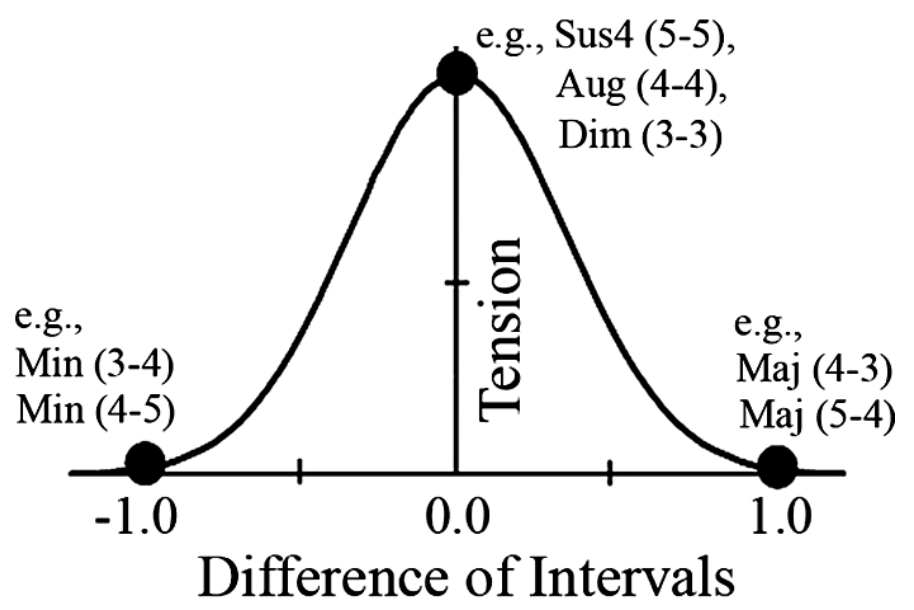# Lateral Music Theory #2: Tension, Instability

Continuing on from the earlier discussion on dissonance, there is another couple of numeric quantities that can help describe how chords are perceived. These are Tension and Instability. Instability matches the empirical perception of 3 note chords.

Tension is a word used to describe the ambiguity of how a chord seems to want to resolve. It is calculated from the relative size of the intervals of a three note combination. Specifically, from all the combinations of 3 tones from all the partials of the 3 fundamental tones.where:

ν is the product of the relative amplitudes of the three tones (0.0 to 1.0),

x and y are the interval sizes of the lower and upper intervals defined in semitones

α = 0.6 determines the steepness of the fall from maximal tension.Total Instability is (I) is calculated from Tension (T) and Dissonance (D)

I = D + δT

where δ = 0.2

When all the partial combination D and T effects are summed in this way, the scores rank the sonority in a similar order to the empirically determined order.

You can calculate your own dissonance, tension, and instability for chords at a web page I wrote a while ago: here.